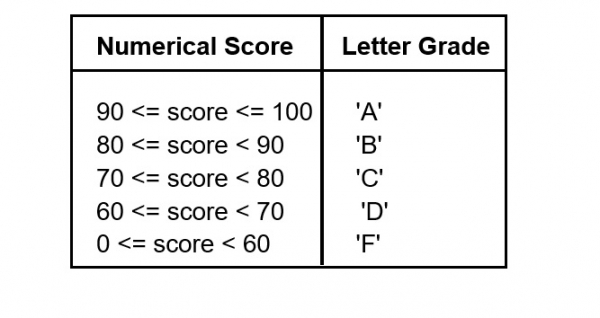We are required to write a function that finds the mean of the three scores passed to it and returns the letter value associated with that grade according to the following table.## Example

const findGrade = (...scores) => {
const {
length
} = scores;
const sum = scores.reduce((acc, val) => acc + val);
const score = sum / length;
if (score >= 90 && score <= 100) {
return 'A';
}
else if (score >= 80 ) {
return 'B';
} else if (score >= 70 ) {
return 'C';
} else if (score >= 60) {
return 'D';
} else{
return 'F';
};
}
console.log(findGrade(92,70,40));

## Output

And the output in the console will be −

F
C
D

Updated on: 23-Nov-2020

176 Views